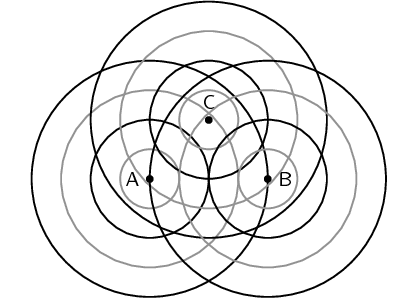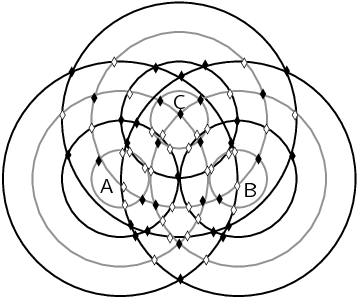Home Practice
For learners and parents For teachers and schools
Textbooks
Full catalogue
Pricing SupportLog in

We think you are located in United States. Is this correct?

# End of chapter exercises

Textbook Exercise 6.2

In the diagram below the peaks of wavefronts are shown by black lines and the troughs by grey lines. Mark all the points where constructive interference between two waves is taking place and where destructive interference is taking place. Also note whether the interference results in a peak or a trough.The solid diamonds represent constructive interference (crests) and the open diamonds represent destructive interference (troughts).For a slit of width $$\text{1 300}$$ $$\text{nm}$$, order the following EM waves from least to most diffracted:

1. green at $$\text{510}$$ $$\text{nm}$$

2. blue at $$\text{475}$$ $$\text{nm}$$

3. red at $$\text{650}$$ $$\text{nm}$$

4. yellow at $$\text{570}$$ $$\text{nm}$$

blue, green, yellow, red

For light of wavelength $$\text{540}$$ $$\text{nm}$$, determine which of the following slits widths results in the maximum and which results in the minimum amount of diffraction

1. $$\text{323} \times \text{10}^{-\text{9}}$$ $$\text{m}$$

2. $$\text{12,47}$$ $$\text{nm}$$

3. $$\text{21,1}$$ $$\text{pm}$$

We know that narrower slits give more diffraction. So we need to determine which is the narrowest slit and which is the widest. $$\text{21,1}$$ $$\text{pm}$$ is the narrowest slit (A $$\text{pm}$$ is $$\text{10}^{-\text{12}}$$ $$\text{m}$$). The widest slit is $$\text{323} \times \text{10}^{-\text{9}}$$ $$\text{m}$$ (this is the same as $$\text{323}$$ $$\text{nm}$$).

So the slit with the maximum diffraction is $$\text{21,1}$$ $$\text{pm}$$.

The slit with the minimum diffraction is $$\text{323}$$ $$\text{nm}$$.

For light of wavelength $$\text{635}$$ $$\text{nm}$$, determine what the width of the slit needs to be to have the diffraction be less than the angle of diffraction in each of these cases:

Water waves at the entrance to a harbour which has a rock barrier with a $$\text{3}$$ $$\text{m}$$ wide opening. The waves have a wavelength of $$\text{16}$$ $$\text{m}$$ wavelength approach the opening straight on.

The angle of diffraction for the water waves is:

\begin{align*} \sin \theta & = \dfrac{m \lambda}{w} \\ & = \dfrac{\text{3}\text{ m}}{\text{16}\text{ m}} \\ \sin \theta & = \text{0,1875} \\ \theta &= \text{10,81} \end{align*}

We need to find a slit width that will give a smaller angle of diffraction for light of $$\text{635}$$ $$\text{nm}$$:

\begin{align*} \sin \theta & = \dfrac{m \lambda}{w} \\ \text{0,1875} & = \dfrac{\text{635} \times \text{10}^{-\text{9}}\text{ m}}{w} \\ w & = \frac{\text{635} \times \text{10}^{-\text{9}}\text{ m}}{\text{0,1875}} \\ &= \text{3,4} \times \text{10}^{-\text{6}}\text{ m} \end{align*}

The slit must be less than $$\text{3,4} \times \text{10}^{-\text{6}}$$ $$\text{m}$$.

Light with a wavelength of $$\text{786} \times \text{10}^{-\text{9}}$$ $$\text{m}$$ strikes a single slit of width $$\text{30} \times \text{10}^{-\text{7}}$$ $$\text{m}$$.

The angle of diffraction is:

\begin{align*} \sin \theta & = \dfrac{m \lambda}{w} \\ & = \dfrac{\text{786} \times \text{10}^{-\text{9}}\text{ m}}{\text{30} \times \text{10}^{-\text{7}}\text{ m}} \\ \sin \theta & = \text{0,262} \\ \theta &= \text{15,18} \end{align*}

We need to find a slit width that will give a smaller angle of diffraction for light of $$\text{635}$$ $$\text{nm}$$:

\begin{align*} \sin \theta & = \dfrac{m \lambda}{w} \\ \text{0,262} & = \dfrac{\text{635} \times \text{10}^{-\text{9}}\text{ m}}{w} \\ w & = \frac{\text{635} \times \text{10}^{-\text{9}}\text{ m}}{\text{0,262}} \\ &= \text{2,4} \times \text{10}^{-\text{6}}\text{ m} \end{align*}

The slit must be less than $$\text{2,4} \times \text{10}^{-\text{6}}$$ $$\text{m}$$.